# The Difference Between a Gate and a Population: Using the Population Manager and considerations for deleting and renaming gates

Background

In Cytobank, the terms population and gate are different and the distinction is important to understand.

A gate is a shape drawn on a plot to encapsulate a set of events, but other than that, it carries no other information about hierarchy or complex cellular identity. An example of a gate might be CD4+. You may know what population this gate corresponds to, but that single gate most certainly does not define CD4+ T cells. The population is defined by many gates in sequence.

In order to build information about hierarchy and to categorize cellular identity from gates, populations are used. A population is simply a collection of gates. In the example above, the population CD4+ T cells could be defined by the gates Scatter AND Live cells AND singlets AND CD3+ AND CD4+. This is the gate set of the population, and after applying all the gates, you will have the events of interest that define that actual biological population of interest. Contrary to popular intuitive believe, the order in which you apply these gates doesn't matter to obtaining the final filtered events.

Since gates and populations are decoupled, it is important to understand how to control which gates a population is defined by. Controlling the gate set definition of a population is also the basis of boolean gating. In Cytobank, the Population Manager is what confers control over populations and the gates that define them.

Introducing the Population Manager and how gate sets become ordered hierarchies

Normally, you define what gates are in a population without knowing it, simply by drawing a gate, activating it, and then drawing the next gate, as is typical in flow analysis. Doing this will result in a population tree that could look like this: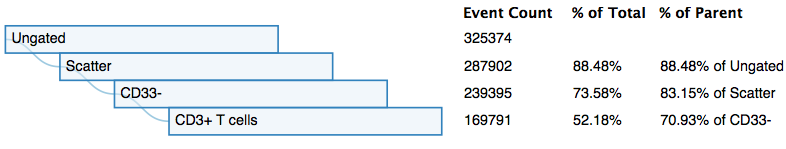(a basic population tree)

When you gate in this serial fashion in Cytobank, a population is created automatically for each gate drawn, and each new population automatically contains all the gates of its parent, plus the one you just drew. This might be more easily understood in the figure below, which shows the correlate population manager for for the population tree above. The image also highlights the anatomy of the population manager: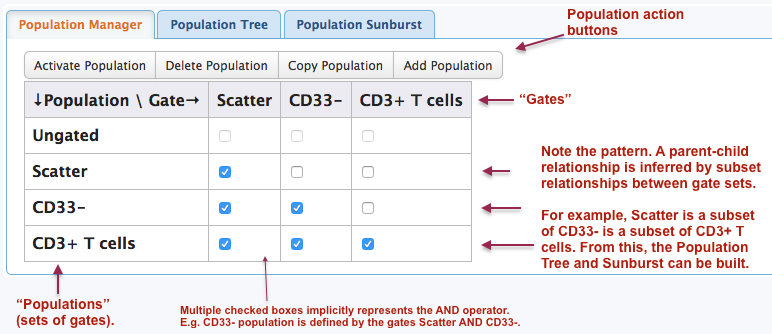(The population manager that represents the Population Tree above. Notes included)

An obvious pattern can be seen where each new population consists of a previous population plus one additional gate. Comparing subset relationships of gate sets between populations is how the population tree hierarchy is determined. Specifically, for a parent-child relationship to exist, one population must be a proper subset of another, as defined by the gates included in the set. In this example, CD33- is the most similar proper subset of CD3+ T cells, and thus it is the immediate parent.

The check boxes in the population manager are used to create the definition of a population. A checked box means to include the gate in the population.

Another example: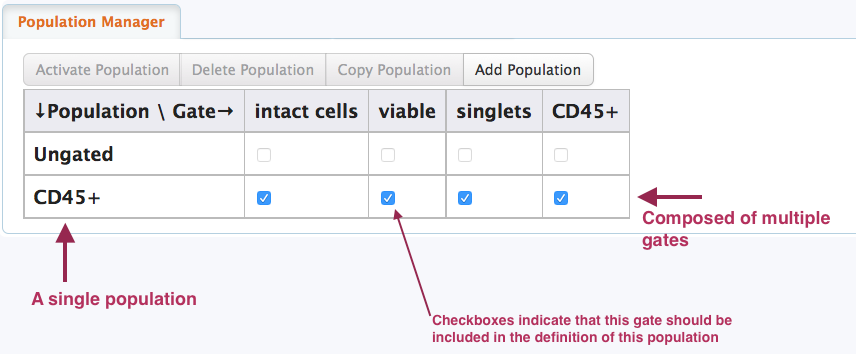(CD45+ population could be intact cells AND viable AND singlets AND CD45+)

Deleting or renaming a gate requires actions in the population manager

Consider this scenario below, where we have drawn a scatter gate, which created a scatter population. We then activated the scatter population, and drew a singlets gate. The resulting population manager and population tree are shown: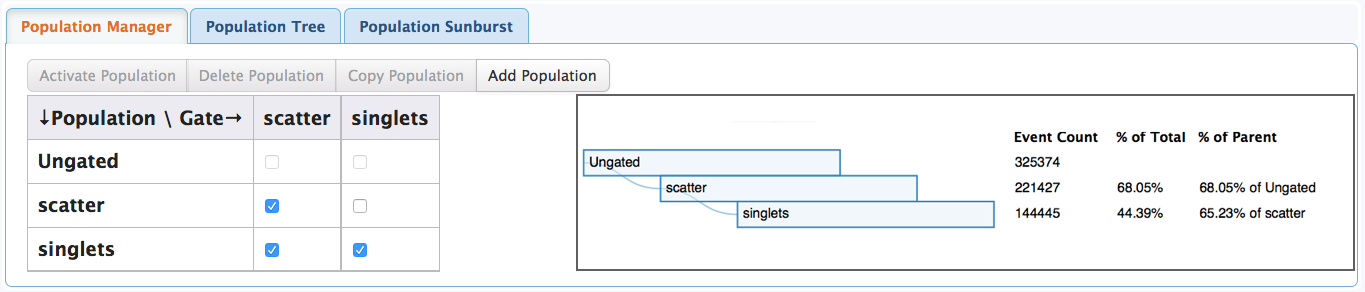As expected, the singlets population is composed of the scatter gate and the singlets gate. It is a child of the scatter population.

But what happens if we delete the singlets gate? Perhaps we drew it on the wrong channel or scales, or maybe we just want to change the gate type. Below is an image of the population manager and population tree right after the gate is deleted.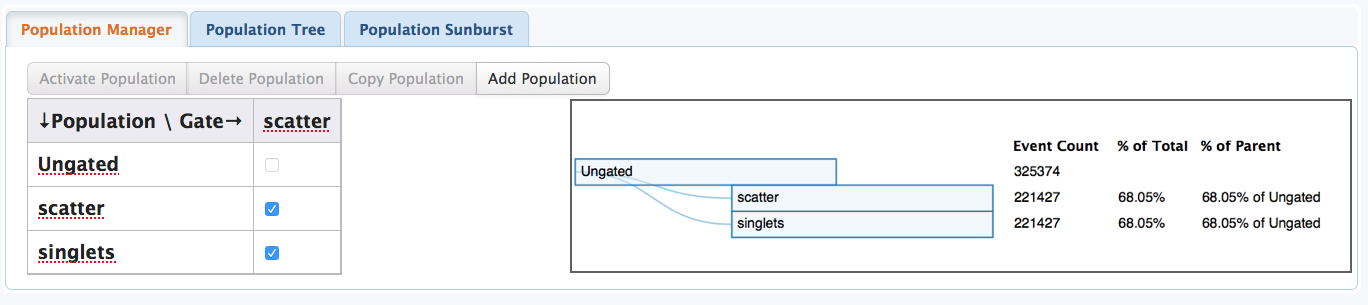(after deleting a gate, you must delete the correlate population)

The singlets population remains in the population manager and population tree because a gate is not a population. Deleting the gate will not delete its correlate population. The singlets population also sits in a different place in the population tree after the deletion because we removed an important defining gate from its definition. Technically, it is now defined as the same population as scatter! This explains why it has the same statistics.

The same principal above applies to renaming gates and then having to rename the correlate population.

Have more questions? Submit a request